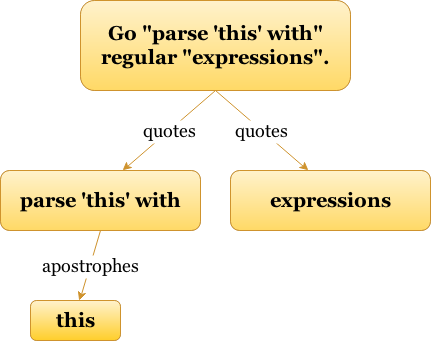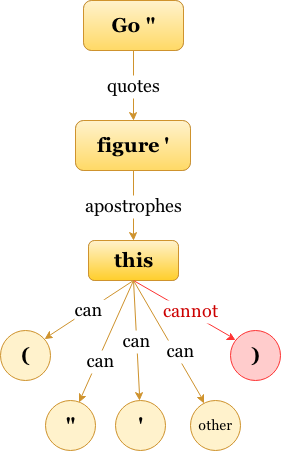Tools
GitHubLibrary

# Lightpath

## A universal recursive data modeller defined by a simple, single-construct grammar

### ( Go “parse ‘this’ with” regular “expressions”. )

````(root)`
`  END? eof`
`  +root "braces" = (`
`    END = )`
`  +root "quotes" = "`
`    END = "`
`  +root "apostrophes" = '`
`    END = '`
`  "other" % [^()"']`
`root````### ( Go “figure ‘this) error’. ”